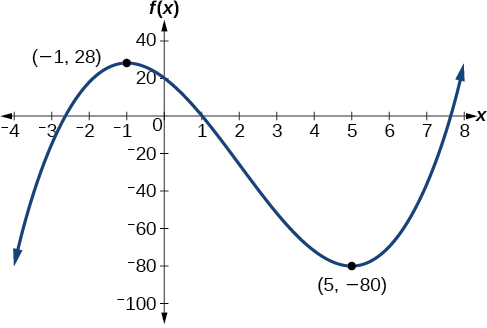# 3.3 Rates of change and behavior of graphs  (Page 4/15)

 Page 4 / 15

## Finding increasing and decreasing intervals on a graph

Given the function $\text{\hspace{0.17em}}p\left(t\right)\text{\hspace{0.17em}}$ in [link] , identify the intervals on which the function appears to be increasing.

We see that the function is not constant on any interval. The function is increasing where it slants upward as we move to the right and decreasing where it slants downward as we move to the right. The function appears to be increasing from $\text{\hspace{0.17em}}t=1\text{\hspace{0.17em}}$ to $\text{\hspace{0.17em}}t=3\text{\hspace{0.17em}}$ and from $\text{\hspace{0.17em}}t=4\text{\hspace{0.17em}}$ on.

In interval notation    , we would say the function appears to be increasing on the interval (1,3) and the interval $\left(4,\infty \right).$

## Finding local extrema from a graph

Graph the function $\text{\hspace{0.17em}}f\left(x\right)=\frac{2}{x}+\frac{x}{3}.\text{\hspace{0.17em}}$ Then use the graph to estimate the local extrema of the function and to determine the intervals on which the function is increasing.

Using technology, we find that the graph of the function looks like that in [link] . It appears there is a low point, or local minimum, between $\text{\hspace{0.17em}}x=2\text{\hspace{0.17em}}$ and $\text{\hspace{0.17em}}x=3,\text{\hspace{0.17em}}$ and a mirror-image high point, or local maximum, somewhere between $\text{\hspace{0.17em}}x=-3\text{\hspace{0.17em}}$ and $\text{\hspace{0.17em}}x=-2.$

Graph the function $\text{\hspace{0.17em}}f\left(x\right)={x}^{3}-6{x}^{2}-15x+20\text{\hspace{0.17em}}$ to estimate the local extrema of the function. Use these to determine the intervals on which the function is increasing and decreasing.

The local maximum appears to occur at $\text{\hspace{0.17em}}\left(-1,28\right),\text{\hspace{0.17em}}$ and the local minimum occurs at $\text{\hspace{0.17em}}\left(5,-80\right).\text{\hspace{0.17em}}$ The function is increasing on $\text{\hspace{0.17em}}\left(-\infty ,-1\right)\cup \left(5,\infty \right)\text{\hspace{0.17em}}$ and decreasing on $\text{\hspace{0.17em}}\left(-1,5\right).$## Finding local maxima and minima from a graph

For the function $\text{\hspace{0.17em}}f\text{\hspace{0.17em}}$ whose graph is shown in [link] , find all local maxima and minima.

Observe the graph of $\text{\hspace{0.17em}}f.\text{\hspace{0.17em}}$ The graph attains a local maximum at $\text{\hspace{0.17em}}x=1\text{\hspace{0.17em}}$ because it is the highest point in an open interval around $\text{\hspace{0.17em}}x=1.$ The local maximum is the $\text{\hspace{0.17em}}y$ -coordinate at $\text{\hspace{0.17em}}x=1,\text{\hspace{0.17em}}$ which is $\text{\hspace{0.17em}}2.$

The graph attains a local minimum at because it is the lowest point in an open interval around $\text{\hspace{0.17em}}x=-1.\text{\hspace{0.17em}}$ The local minimum is the y -coordinate at $\text{\hspace{0.17em}}\text{\hspace{0.17em}}x=-1,\text{\hspace{0.17em}}\text{\hspace{0.17em}}$ which is $\text{\hspace{0.17em}}\text{\hspace{0.17em}}-2.$

## Analyzing the toolkit functions for increasing or decreasing intervals

We will now return to our toolkit functions and discuss their graphical behavior in [link] , [link] , and [link] .

## Use a graph to locate the absolute maximum and absolute minimum

There is a difference between locating the highest and lowest points on a graph in a region around an open interval (locally) and locating the highest and lowest points on the graph for the entire domain. The $\text{\hspace{0.17em}}y\text{-}$ coordinates (output) at the highest and lowest points are called the absolute maximum and absolute minimum , respectively.

To locate absolute maxima and minima from a graph, we need to observe the graph to determine where the graph attains it highest and lowest points on the domain of the function. See [link] .

Not every function has an absolute maximum or minimum value. The toolkit function $\text{\hspace{0.17em}}f\left(x\right)={x}^{3}\text{\hspace{0.17em}}$ is one such function.

## Absolute maxima and minima

The absolute maximum    of $\text{\hspace{0.17em}}f\text{\hspace{0.17em}}$ at $\text{\hspace{0.17em}}x=c\text{\hspace{0.17em}}$ is $\text{\hspace{0.17em}}f\left(c\right)\text{\hspace{0.17em}}$ where $\text{\hspace{0.17em}}f\left(c\right)\ge f\left(x\right)\text{\hspace{0.17em}}$ for all $\text{\hspace{0.17em}}x\text{\hspace{0.17em}}$ in the domain of $\text{\hspace{0.17em}}f.$

The absolute minimum    of $\text{\hspace{0.17em}}f\text{\hspace{0.17em}}$ at $\text{\hspace{0.17em}}x=d\text{\hspace{0.17em}}$ is $\text{\hspace{0.17em}}f\left(d\right)\text{\hspace{0.17em}}$ where $\text{\hspace{0.17em}}f\left(d\right)\le f\left(x\right)\text{\hspace{0.17em}}$ for all $\text{\hspace{0.17em}}x\text{\hspace{0.17em}}$ in the domain of $\text{\hspace{0.17em}}f.$

## Finding absolute maxima and minima from a graph

For the function $\text{\hspace{0.17em}}f\text{\hspace{0.17em}}$ shown in [link] , find all absolute maxima and minima.

Observe the graph of $\text{\hspace{0.17em}}f.\text{\hspace{0.17em}}$ The graph attains an absolute maximum in two locations, $\text{\hspace{0.17em}}x=-2\text{\hspace{0.17em}}$ and $\text{\hspace{0.17em}}x=2,\text{\hspace{0.17em}}$ because at these locations, the graph attains its highest point on the domain of the function. The absolute maximum is the y -coordinate at $\text{\hspace{0.17em}}x=-2\text{\hspace{0.17em}}$ and $\text{\hspace{0.17em}}x=2,\text{\hspace{0.17em}}$ which is $\text{\hspace{0.17em}}16.$

The graph attains an absolute minimum at $\text{\hspace{0.17em}}x=3,\text{\hspace{0.17em}}$ because it is the lowest point on the domain of the function’s graph. The absolute minimum is the y -coordinate at $\text{\hspace{0.17em}}x=3,$ which is $-10.$

#### Questions & Answers

write down the polynomial function with root 1/3,2,-3 with solution
if A and B are subspaces of V prove that (A+B)/B=A/(A-B)
write down the value of each of the following in surd form a)cos(-65°) b)sin(-180°)c)tan(225°)d)tan(135°)
Prove that (sinA/1-cosA - 1-cosA/sinA) (cosA/1-sinA - 1-sinA/cosA) = 4
what is the answer to dividing negative index
In a triangle ABC prove that. (b+c)cosA+(c+a)cosB+(a+b)cisC=a+b+c.
give me the waec 2019 questions
the polar co-ordinate of the point (-1, -1)
prove the identites sin x ( 1+ tan x )+ cos x ( 1+ cot x )= sec x + cosec x
tanh`(x-iy) =A+iB, find A and B
B=Ai-itan(hx-hiy)
Rukmini
what is the addition of 101011 with 101010
If those numbers are binary, it's 1010101. If they are base 10, it's 202021.
Jack
extra power 4 minus 5 x cube + 7 x square minus 5 x + 1 equal to zero
the gradient function of a curve is 2x+4 and the curve passes through point (1,4) find the equation of the curve
1+cos²A/cos²A=2cosec²A-1
test for convergence the series 1+x/2+2!/9x3ByByByByBy